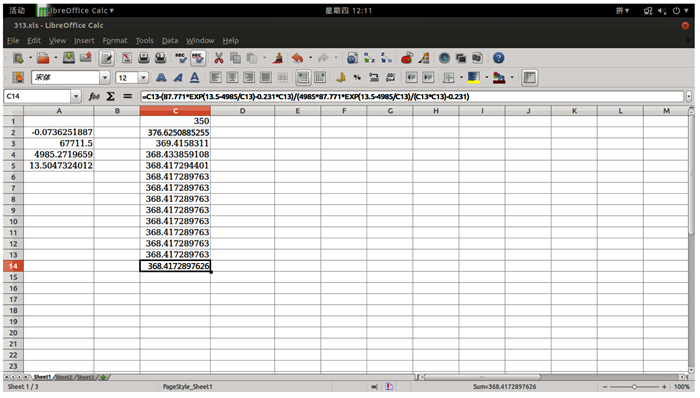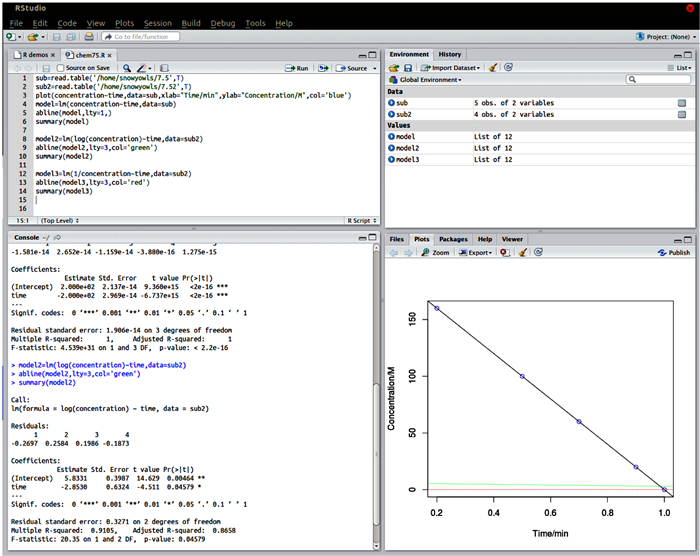## An Attempt to Lead Students Solving Chemical Principle Exercises by Free Software in Recitation ClassAbstract

Free software, such as Scilab, LiberOffice and R, were applied to solve chemical principle exercises, and the solving procession was demonstrated in recitation class. Such attempts have trained ability of undergraduate students to solve practical problems by scientific methods, and led students to focus on the application of free software in scientific computation.

Keywords： Chemical principle ; Free software ; Scientific computation

CUI Wei. An Attempt to Lead Students Solving Chemical Principle Exercises by Free Software in Recitation Class. University Chemistry[J], 2017, 32(3): 63-69 doi:10.3866/PKU.DXHX201609011

## 1 背景与意义

### 2.1 应用数值分析软件Scilab解题

$\left\{ \begin{gathered} pV = nRT \hfill \\ \ln \left( {\frac{p}{{{p_0}}}} \right) = \frac{{\Delta {H_{{\text{vap}}}}}}{R}\left( {\frac{1}{{{T_0}}} - \frac{1}{T}} \right) \hfill \\ \end{gathered} \right.$

$\left\{ \begin{gathered} pV = nRT \hfill \\ p = {p_0}\exp \left( {\frac{{\Delta {H_{{\text{vap}}}}}}{R}\left( {\frac{1}{{{T_0}}} - \frac{1}{T}} \right)} \right) \hfill \\ \end{gathered} \right.$

${p_0}\exp \left( {\frac{{\Delta {H_{{\text{vap}}}}}}{R}\left( {\frac{1}{{{T_0}}} - \frac{1}{T}} \right)} \right) - \frac{{nRT}}{V} = 0$

Scilab中求解非线方程组的命令为fsolve，可以求得本习题中超越方程的数值解。求解程序如下：

Scilab中求解非线方程组的命令为fsolve，可以求得本习题中超越方程的数值解。求解程序如下：

function y=fct (x)

y=198.5*exp (12.64-4970/x)-0.231*x;

endfunction

//定义函数

fsolve (393, fct)

//从T=393开始迭代求解方程

fplot2d ([1:393], fct)

a=gca ();

a.grid=[5 5];

//绘制函数图线

### 图1### 2.2 应用办公软件LibreOffice解题

LibreOffice的前身是著名开源办公软件OpenOffic.org，在母公司被Oracle收购后，由文件基金会独立开发，是Microsoft Office的开源替代软件。其中的电子表格软件LibreOfficeCalc可以开展基于电子表格的迭代计算。

xn的取值将随迭代逼近方程f(x)=0的解。应用电子表格软件开展迭代计算求解本题温度方程的步骤如下：

=C1-(87.771*EXP (13.5-4985/C1)-0.231*C1)/(4985*87.771*EXP (13.5-4985/C1)/(C1*C1)-0.231)

### 图2### 2.3 应用统计软件R解题

 t/min 0 20 60 100 160 c(S)/(mol∙dm-3) 1 0.9 0.7 0.5 0.2

### 图3plot (concentration~time, data=sub, xlab="Time/min", ylab="Concentration/M", col=′blue′)

#读取原始数据

model=lm (concentration~time, data=sub)

abline (model, lty=1, )

summary (model)

#一级动力学回归拟合,并绘制黑色实线回归方程曲线

model2=lm (log (concentration)~time, data=sub2)

abline (model2, lty=3, col=′green′)

summary (model2)

#二级动力学回归拟合,并绘制红色虚线回归方程曲线

model3=lm (1/concentration~time, data=sub2)

abline (model3, lty=3, col=′red′)

summary (model3)

#三级动力学回归拟合,并绘制绿色圆点虚线回归方程曲线

## 参考文献 原文顺序 文献年度倒序 文中引用次数倒序 被引期刊影响因子

Levin, R. C. The Work of the University; Yale University Press:New Haven, 2003.

Cossairt T. J. ; Grubbs W. T. J. Chem. Educ 2011, 88 (6), 841.

Francoia J. P. ; Vial L J. Chem. Educ 2016, 93 (6), 1137.

Libman D. ; Huang L. J. Chem. Educ 2013, 90 (3), 320.

Foundation, F. S. What is Free Software? The Free Software Definition.[2016-08-16]. https://www.gnu.org/philosophy/free-sw.en.html.

DiBona, C.; Ockman, S.; Stone, M. Open Sources:Voices from the Open Source Revolution. 1st ed.; 1999, Beijing; O'Reilly:Sebastopol, CA.

DiBona, C.; Cooper, D.; Stone, M. Open Sources 2.0:The Continuing Evolution, 1st ed.; 2006, Beijing; O'Reilly:Sebastopol, CA.

Enterprises, S. Scilab:Free and Open Source Software for Numerical Computation, 2012.

Project, T. L. LibreOffice; 2015, The Document Foundation.

Team, R. C. R:A Language and Environment for Statistical Computing:R Foundation for Statistical Computing:Vienna, Austria, 2016.

Team, R. RStudio:Integrated Development Environment for R; RStudio, Inc.; Boston, MA, 2015.

/

 〈〉